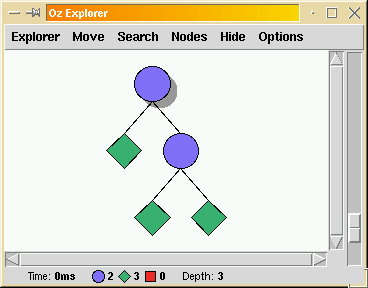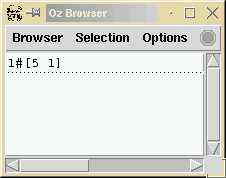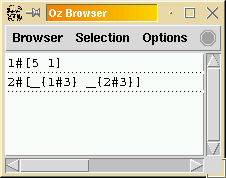6.6 Search Predicates

A typical way to solve a CSP in Oz, is to express this CSP as a predicate and invoke the Explorer [Sch97a] [Sch99] with this predicate as an argument.

{ExploreAll SimpleProblem}

We explain now how to write such a predicate. It always follows the same pattern.

declare
proc {SimpleProblem Solution}
%% declare variables
X Y
in
%% representation of solution
Solution = [X Y]

%% pose constraints of CSP
X::1#9
Y::1#9
X+2*=: 7

%% distribution strategy
{FD.distribute ff [X Y]}
end

SimpleProblem is a predicate that defines what is a Solution: a solution is a list [X Y] of two integers satisfying the equation X+2*=: 7. It invokes the predefined distribution strategy {FD.distribute ff [X Y]}, where ff means first-fail i.e. ``choose the variable with the fewer remaining possible values, and then try each one of these values in increasing order''.

{ExploreAll SimpleProblem} produces the following search tree in the Explorer:clicking on the first green diamond (a solution) shows the following in the browser:We can also click on the second blue circle (a choice point) to observe the partial information at that point:For a thorough tutorial on constraint programming in Oz, we recommend again to read [SS99].

Denys Duchier
Version 1.2.0 (20010221)